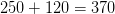# SSAT Upper Level Math : SSAT Upper Level Quantitative (Math)

## Example Questions

### Example Question #1611 : Ssat Upper Level Quantitative (Math)

An arithmetic sequence begins as follows: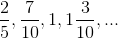Give the thirty-third term of this sequence.

The correct answer is not given among the other four responses.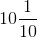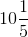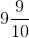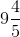The correct answer is not given among the other four responses.

Explanation:

Theth term of an arithmetic sequence with initial term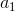and common differenceis defined by the equation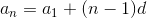.

The initial term in the given sequence is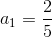;

the common difference is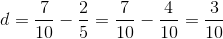.

We are seeking term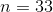.

Therefore,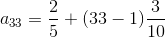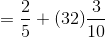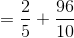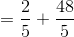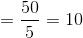,

which is not among the choices.

### Example Question #1 : How To Find The Next Term In An Arithmetic Sequence

What is the value of x in the sequence below?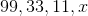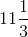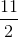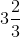Explanation:

In this sequence, each subsequent number is equal to one third of the preceding number.

One third of 11 is equal to: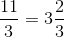Therefore, the correct answer is:### Example Question #2 : How To Find The Next Term In An Arithmetic Sequence

Find the next term of the arithmetic sequence: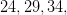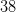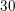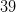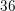Explanation:

The common difference for this sequence is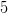. To find the next number in the sequence, addto the last given number.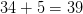### Example Question #3 : How To Find The Next Term In An Arithmetic Sequence

Find the next term of this arithmetic sequence: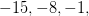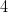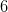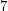Explanation:

The common difference for this sequence is. Add this to the last given term to find the next one.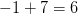### Example Question #4 : How To Find The Next Term In An Arithmetic Sequence

Find the next term of the arithmetic sequence: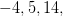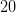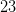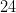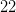Explanation:

The common difference is. Add this to the last given term to find the next term.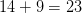### Example Question #5 : How To Find The Next Term In An Arithmetic Sequence

Find the next term of the arithmetic sequence: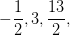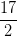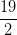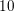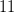Explanation:

The common difference is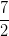. Add this to the last given term to find the next term.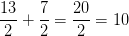### Example Question #6 : How To Find The Next Term In An Arithmetic Sequence

Find the next term of the arithmetic sequence: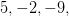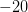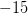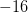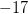Explanation:

The common difference is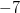. Add this to the last given term to find the next term.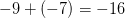### Example Question #7 : How To Find The Next Term In An Arithmetic Sequence

Find the next term of the arithmetic sequence: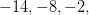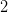Explanation:

The common difference is. Add this to the last given term to find the next term.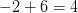### Example Question #8 : How To Find The Next Term In An Arithmetic Sequence

Find the next term of the arithmetic sequence: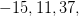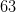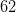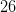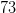Explanation:

The common difference is. Add this to the last given term to find the next term.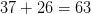### Example Question #9 : How To Find The Next Term In An Arithmetic Sequence

Find the next term of the arithmetic sequence: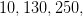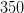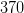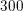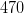The common difference is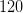. Add this to the last given term to find the next term.